# Chapter 10: Basic Geometrical Concepts Exercise 10.3

### Question: 1

Draw rough diagrams to illustrate the following:

i) Open curve

ii) Closed curve

### Solution:

i) Open curve: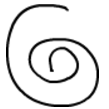ii) Closed curve:### Question: 2

Classify the following curves as open or closed?

i)ii)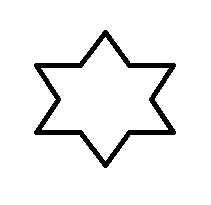iii)iv)v)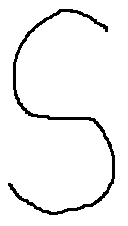vi)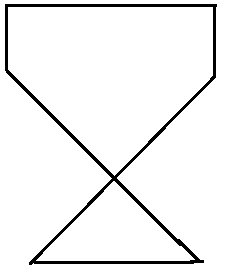i) Open

ii) Closed

iii) Closed

iv) Open

v) Open

vi) Closed

### Question: 3

Draw a polygon and shade its interior. Also draw its diagonals, if any

### Solution:

ABCD is a polygon and AC and BD are its two diagonals.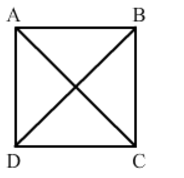### Question: 4

Illustrate, if possible, each one of the following with a rough diagram:

i) A closed curve that is not a polygon

ii) An open curve made up entirely of line segments

iii) A polygon with two sides

### Solution:

i) A circle is a simple closed curve but not a polygon. A polygon has line segments, but a circle has only curve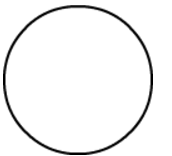ii) Rough diagram of an open curve made up entirely of line segments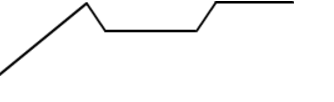iii) A polygon with two sides is not possible.

### Course Features

• Video Lectures
• Revision Notes
• Previous Year Papers
• Mind Map
• Study Planner
• NCERT Solutions
• Discussion Forum
• Test paper with Video Solution

r